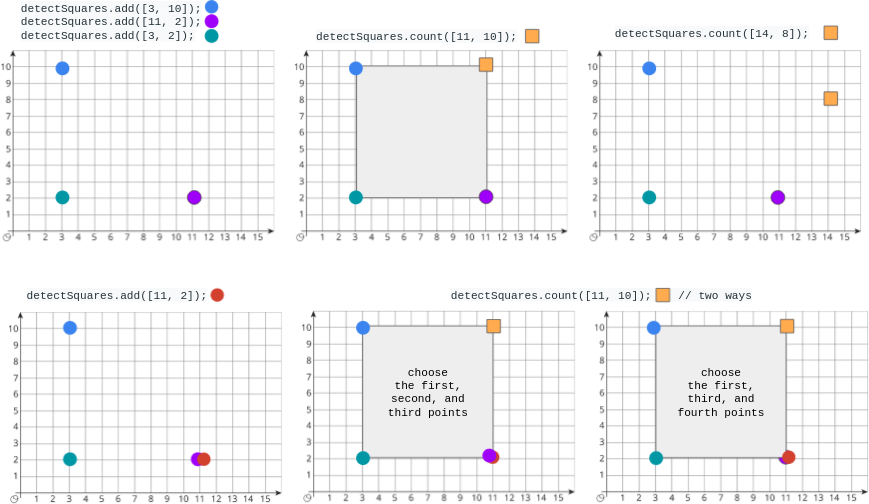Formatted question description: https://leetcode.ca/all/2013.html

# 2013. Detect Squares (Medium)

You are given a stream of points on the X-Y plane. Design an algorithm that:

• Adds new points from the stream into a data structure. Duplicate points are allowed and should be treated as different points.
• Given a query point, counts the number of ways to choose three points from the data structure such that the three points and the query point form an axis-aligned square with positive area.

An axis-aligned square is a square whose edges are all the same length and are either parallel or perpendicular to the x-axis and y-axis.

Implement the DetectSquares class:

• DetectSquares() Initializes the object with an empty data structure.
• void add(int[] point) Adds a new point point = [x, y] to the data structure.
• int count(int[] point) Counts the number of ways to form axis-aligned squares with point point = [x, y] as described above.

Example 1:Input
[[], [[3, 10]], [[11, 2]], [[3, 2]], [[11, 10]], [[14, 8]], [[11, 2]], [[11, 10]]]
Output
[null, null, null, null, 1, 0, null, 2]

Explanation
DetectSquares detectSquares = new DetectSquares();
detectSquares.count([11, 10]); // return 1. You can choose:
//   - The first, second, and third points
detectSquares.count([14, 8]);  // return 0. The query point cannot form a square with any points in the data structure.
detectSquares.add([11, 2]);    // Adding duplicate points is allowed.
detectSquares.count([11, 10]); // return 2. You can choose:
//   - The first, second, and third points
//   - The first, third, and fourth points


Constraints:

• point.length == 2
• 0 <= x, y <= 1000
• At most 5000 calls in total will be made to add and count.

## Solution 1. Enumerate seen x values

Intuition: For query (x, y), we try each x values we’ve seen, say x1, as the x value of the other vertical edge. Because we want to form squares, there are only two square candidates possible.

Algorithm:

Given query (x, y), we try each x1 values we’ve seen. Another point must be (x1, y).

Since the side length d = abs(x - x1), the y value of other horizontal side must be either y1 = y + d or y2 = y - d.

So the answer is count(x1, y) * [ count(x, y1) * count(x1, y1) + count(x, y2) * count(x1, y2) ].

// OJ: https://leetcode.com/problems/detect-squares/
// Time:
//      DetectSquares: O(1)
//      count: O(U) where U is the number of unique x values
// Space: O(N)
class DetectSquares {
unordered_map<int, int> cnt;
unordered_set<int> xs;
inline int key(int x, int y) {
return 10000 * x + y;
}
inline int count(int x, int y) {
int k = key(x, y);
return cnt.count(k) ? cnt[k] : 0;
}
public:
DetectSquares() {}
void add(vector<int> p) {
cnt[key(p, p)]++;
xs.insert(p);
}
int count(vector<int> p) {
int x = p, y = p, ans = 0;
for (int x1 : xs) {
if (x1 == x) continue;
int c = count(x1, y), d = abs(x - x1), y1 = y - d, y2 = y + d;
if (c == 0) continue;
ans += c * (count(x, y1) * count(x1, y1) + count(x, y2) * count(x1, y2));
}
return ans;
}
};